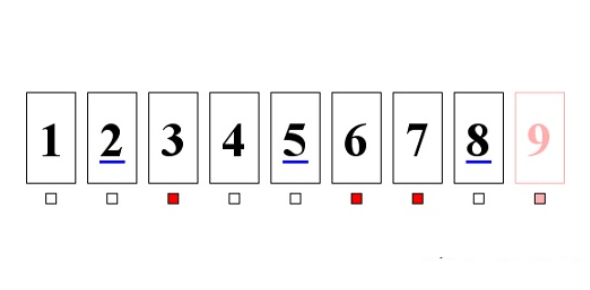# Arithmetic Sequence And Series Quiz!

10 Questions | Total Attempts: 1018SettingsCreate your own QuizAre you experienced with arithmetic sequence and series? An arithmetic sequence is a sequence in which each term is discovered by adding the same value to the previous term. It is a sequence of numbers where the difference between the successive terms is constant. Finding a sequence depends on whether the sequence is arithmetic or geometric. This quiz will tell you if your mathematical knowledge adds up or not.

• 1.
Find the common difference in the Arithmetic Sequence 3,3/14, 7/2, 15/4
• A.

1/4

• B.

3/4

• C.

5/2

• D.

4

• 2.
What is the sum of all odd integers between 8 and 26?
• A.

153

• B.

151

• C.

149

• D.

148

• 3.
If three arithmetic means are inserted between 11 and 39, find the 2nd arithmetic mean.
• A.

46

• B.

32

• C.

25

• D.

18

• 4.
Which term of the Arithmetic Sequence 4,1,-2,-5,... is 29?
• A.

9th term

• B.

10th term

• C.

11th term

• D.

12th term

• 5.
The 1st term of an arithmetic sequence is 2 while the 18th term is 87. Find the common difference of the sequence.
• A.

7

• B.

6

• C.

5

• D.

3

• 6.
Which of the following is the sum of all the multiples of 3 from 15 to 48?
• A.

315

• B.

360

• C.

378

• D.

396

• 7.
What is the nth term of the arithmetic sequence 7,9,11,13,15,17,....?
• A.

3n+4

• B.

2n+5

• C.

4n+3

• D.

N+2

• 8.
Find p so that the numbers 7p+2,5p+12,2p-1,... form an arithmetic sequence.
• A.

-8

• B.

-5

• C.

-13

• D.

-23

• 9.
Glenn bought a car for Php 60,000. The yearly depreciation of his car is 10% of its value at the start of the year. What is its value after 4 years?
• A.

Php 437,400

• B.

Php 438,000

• C.

Php 393,660

• D.

Php 378,000

• 10.
Kelly is saving her money to buy a car. She has Php 50,000 and she plans to save Php 3,750 per week from her job as a call center manager. How much will Kelly have saved after 8 weeks?
• A.

Php 76,000

• B.

Php 76,250

• C.

Php 76,500

• D.

Php 76,750

Related TopicsBack to top
×

Wait!
Here's an interesting quiz for you.